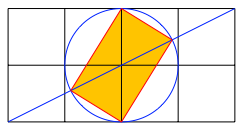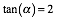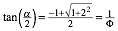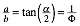Hans Walser, 

The Golden Rectangle in a Square Lattice

# 1        First Example

## 1.1      The Golden RectangleFig. 1: Golden Rectangle

## 1.2      Proof

We use the labeling of Figure 2.Fig. 2: Labeling

First we have. Sincewe get:Moreover we have:Hence we have a Golden Rectangle.

# 2        Second Example

## 2.1      The Golden RectangleFig. 3: The Golden Rectangle

## 2.2      ProofFig. 4: Labeling

Using the labeling of Figure 4, we get againand the proof goes according to the first example.